## Sunday, January 8, 2012

### Sparse Snapshots in DAX / BISM Tabular

SQL Server 2012/Denali | PowerPivot

In my last post I wrote about using delta values instead of full snapshots. However, the amount of data is identical if we using a sparse snapshots instead. For this purpose, the source data from my last post has just to be converted to absolute values as shown below:

 Only delta values Only snapshots (changes)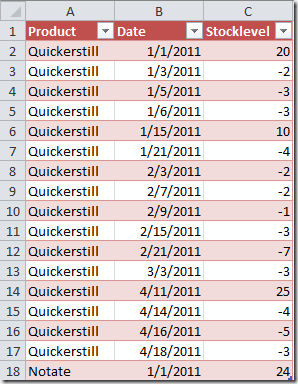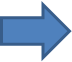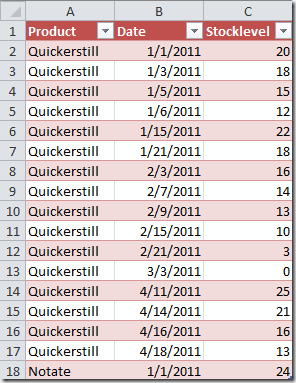So the amount of data is not really changed. But with the sparse snapshots the computations get a lot more difficult. If we have delta values (left table) we can simply sum up all deltas to a given date and this works for all of our related tables (for example products). With sparse snapshots (right table) we have to find out the last value per product and then add the results up to the total, so we have to do a calculation per product before.

Before we get to the calculation, here is the very simple source data model that I used: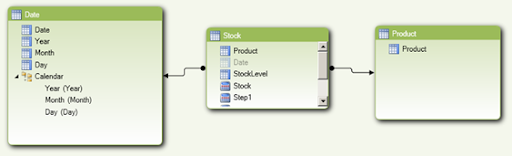It took me some time to figure out the following solution and I’m pretty sure that there must be an easier method. So feel free to experiment and write comments.

Here is the final code:

Stock:=
SumX(
Summarize(
'Product'
,  'Product'[Product]
, "LastStock"
, calculate(
Sum('Stock'[StockLevel])
LastDate('Date'[Date])
,-floor(
LastDate('Date'[Date])
-
MaxX(
Filter(
Summarize(
DatesBetween('Date'[Date], date(2000,1,1), LastDate('Date'[Date]))
,'Date'[Date]
, "X"
,  calculate(Sum('Stock'[StockLevel]), ALLEXCEPT('Date', 'Date'[Date]))
)
, not isblank([X])
)
, 'Date'[Date]
)
, 1
)
,DAY
)
)
)
,
[LastStock]
)

This is how are data looks like without the computation from above: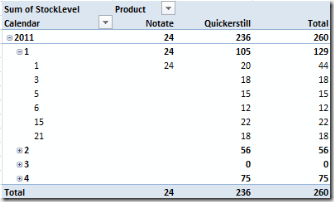Since the data is sparse we only see the stock at dates where there are changes. For the aggregation I used the Sum function (which doesn’t make much sense here for the date aggregates) and you also see that the row total over the products only takes products into account when there are changes.

And here is the resulting Excel pivot table using the calculation from above: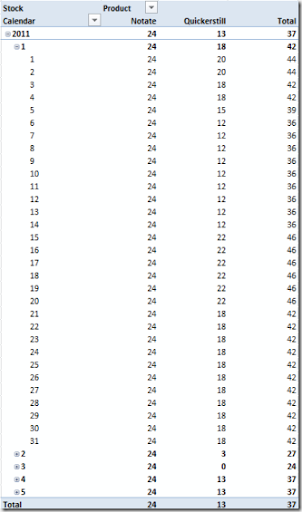As you can see the value for Quickerstill starts with 20 then drops to 18 at January 3, then to 15 at January 5 etc. Also the totals are correct now.

The remaining part of this post is about the formula from above, so it’s up to you to decide if you want to continue reading. The most important point here is that the calculation is much easier when working with delta rows or delta rows with intermediate snapshots (for example each first day of a month, quarter, year).

Details of the calculation

The first question is about the way we’re doing the calculation. Wouldn’t it be easier and faster to have them on the rows and therefore persisted (is ‘persisted’ the right term when talking about an in-memory database??)? Well, if you look at the screenshots above, the only reason we’re doing all this is because we need calculated values on “rows” that do not exist. In the last screenshot there is no row for January 31, but the value has to be computed: For Notate it’s the value of January 1 and for Quickerstill it’s the value of January 21. So we have to use a calculated measure in the model.

To makes things easier here, let’s start with a single product (Quickerstill). I want to calculate the last date for which I have a value. To do this I filter the date range from a very early date (2000/1/1 here) to the last date in the current context to those values, where the sum of the stock amount (any aggregate would do) is not blank, then take the biggest date (max function). This is the code:

Step1:=
MaxX(
Filter(
Summarize(
DatesBetween('Date'[Date], date(2000,1,1), LastDate('Date'[Date]))
,'Date'[Date]
, "X"
,  calculate(Sum('Stock'[StockLevel]), ALLEXCEPT('Date', 'Date'[Date]))
)
, not isblank([X])
)
, 'Date'[Date]
)

If I include the original measure and this calculation in a pivot table, this is the result: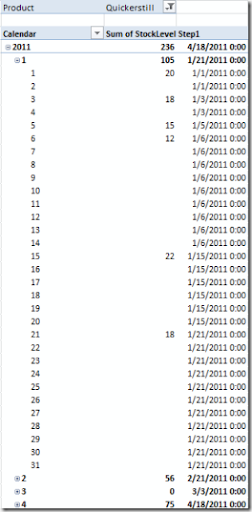For each day our calculation returns the last date with data. For example the calculated date for January 12 is January 6 as this was the last day with data before January 12. It seems we are already close to the solution but please keep in mind that the calculation from above would not work for more than one product (for example if the products are not filtered) as the date for each product has to be different. If we filter the pivot table from above for product Notate, the results would look totally different. Here are the first rows: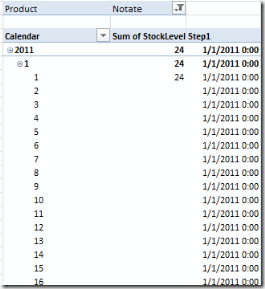But let’s ignore this for a second and keep the filter on Quickerstill. The next task would be to calculate the stock level at the calculated date. This sounds easy and the following formula was my first approach:

Step2 (with error):=
calculate(
Sum('Stock'[StockLevel])
,MaxX(
Filter(
Summarize(
DatesBetween('Date'[Date], date(2000,1,1), LastDate('Date'[Date]))
,'Date'[Date]
, "X"
,  calculate(Sum('Stock'[StockLevel]), ALLEXCEPT('Date', 'Date'[Date]))
)
, not isblank([X])
)
, 'Date'[Date]
)
)

The code in blue is the exactly the code from above which gives the filter context for the calculation of the sum of the stock level values. However, this results in an error:

Semantic Error: A function ‘MAXX‘ has been used in a True/False expression that is used as a table filter expression. This is not allowed.

I love the ‘This is not allowed’ here. Actually there is no reason why it isn’t and I’m not feeling like I’m doing something illegal here. Maybe it’s also just a limitation of the CTP 3 beta release that I am currently working with. I also tried to wrap the MaxX function in a DateAdd with 0 days but this is also ‘not allowed’.

But there is a way which can be found after some time of experimenting. I take the number of days between the last date from the context and the date from the calculation in blue and use this result to correct my last date. Sounds confusing? Let’s start with the number of days. Here is the next step of the calculation:

Step2:=
floor(
LastDate('Date'[Date])
-

MaxX(
Filter(
Summarize(
DatesBetween('Date'[Date], date(2000,1,1), LastDate('Date'[Date]))
,'Date'[Date]
, "X"
,  calculate(Sum('Stock'[StockLevel]), ALLEXCEPT('Date', 'Date'[Date]))
)
, not isblank([X])
)
, 'Date'[Date]
)

, 1
)

Again the code in blue is the last date calculation from above (step 1). Let’s take a look at the result of this calculation: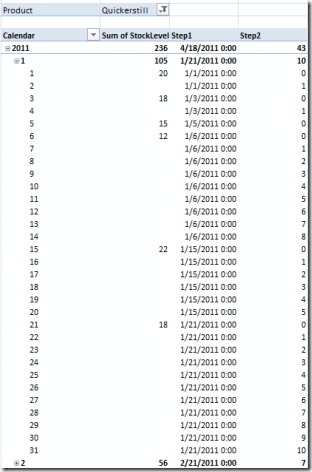As you see, the new calculation tells us, how many days we have to go back in time to find a value. For example for January 14 we get a value of 8 meaning we have to go back 8 days to January 6 to find a value. Now we can wrap this in the calculation of the stock value and for some reason that I don’t understand, this is not illegal anymore although I’m doing exactly the same as I did before. Here is the calculation:

Step3:=
calculate(
Sum('Stock'[StockLevel])
LastDate('Date'[Date])
,-floor(
LastDate('Date'[Date])
-
MaxX(
Filter(
Summarize(
DatesBetween('Date'[Date], date(2000,1,1), LastDate('Date'[Date]))
,'Date'[Date]
, "X"
,  calculate(Sum('Stock'[StockLevel]), ALLEXCEPT('Date', 'Date'[Date]))
)
, not isblank([X])
)
, 'Date'[Date]
)
, 1
)
,DAY
)
)

Again the code from the last step is colored blue (step 2). Now we’re pretty close to the final formula. Let’s check the result when including both products: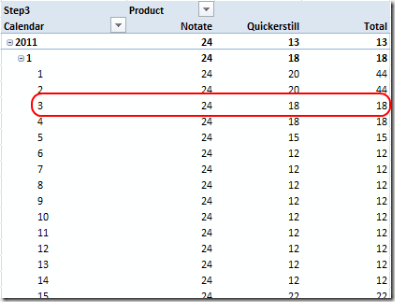Now the result for each product is already working correctly. But the total is still not correct as you can see from the line marked in red. For January 2 the last date with data is the same for both products (January 1), therefore the value is correct. But for January 3 there are different days for the last stock value, so we only see one product in the total.

However, the remaining part is not difficult. We simply summarize (group) by product and take the sum. Again, the code in blue is the last code from the step before (step 3):

Stock:=
SumX(
Summarize(
'Product'
,  'Product'[Product]
, "LastStock"
, calculate(
Sum('Stock'[StockLevel])
LastDate('Date'[Date])
,-floor(
LastDate('Date'[Date])
-
MaxX(
Filter(
Summarize(
DatesBetween('Date'[Date], date(2000,1,1), LastDate('Date'[Date]))
,'Date'[Date]
, "X"
,  calculate(Sum('Stock'[StockLevel]), ALLEXCEPT('Date', 'Date'[Date]))
)
, not isblank([X])
)
, 'Date'[Date]
)
, 1
)
,DAY
)
)
)
,
[LastStock]
)

Now, this does the trick and we end up with the final screenshot as shown at the beginning of this post.

If you like you can play with the formula by downloading the sample work book here (right click on the link, then choose ‘Save as…’). You will need the PowerPivot Add-In CTP 3 or later in order to open the workbook.

1.Hi, thank you for the post
2.